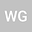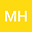Determining lowest excitation energy using the Franck-Hertz experiment
••• William P. Gammel,
William P. Gammel

Corresponding Author:[email protected]

Author ProfileThe lowest excitation energy of the elements Neon, Mercury, and Argon was determined by analyzing the fundamental properties of the signal structure in the Franck-Hertz experiment. In order to accurately determine the lowest excitation energy a new method proposed in \cite{Rapior_2006} was employed. The main idea is that the spacings between the minima in the Franck Hertz curve increase linearly due to the additional acceleration over the mean free path. Therefore a linear fit was applied to graphs of spacings $$\Delta E$$ versus minimum order $$n$$. The fit estimated the lowest excitation energies of Neon ($$19.54\pm 1.48eV$$), Mercury ($$4.72\pm.25eV$$), and Argon ($$11.36\pm.38eV$$) accurately within experimental uncertainty.